The VARMAX Procedure

VARMAX Model

Subsections:

The vector autoregressive moving-average model with exogenous variables is called the VARMAX(p,q,s) model. The form of the model can be written as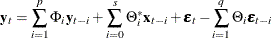where the output variables of interest,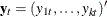, can be influenced by other input variables,, which are determined outside of the system of interest. The variables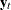are referred to as dependent, response, or endogenous variables, and the variables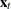are referred to as independent, input, predictor, regressor, or exogenous variables. The unobserved noise variables,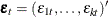, are a vector white noise process.

The VARMAX(p,q,s) model can be written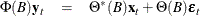where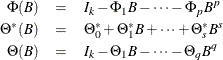are matrix polynomials in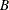in the backshift operator, such that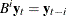, the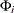and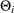are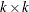matrices, and the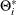are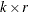matrices.

•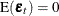,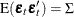, which is positive-definite, and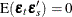for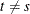.

• For stationarity and invertibility of the VARMAX process, the roots of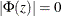and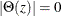are outside the unit circle.

• The exogenous (independent) variablesare not correlated with residuals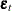,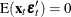. The exogenous variables can be stochastic or nonstochastic. When the exogenous variables are stochastic and their future values are unknown, forecasts of these future values are needed to forecast the future values of the endogenous (dependent) variables. On occasion, future values of the exogenous variables can be assumed to be known because they are deterministic variables. The VARMAX procedure assumes that the exogenous variables are nonstochastic if future values are available in the input data set. Otherwise, the exogenous variables are assumed to be stochastic and their future values are forecasted by assuming that they follow the VARMA(p,q) model, prior to forecasting the endogenous variables, where p and q are the same as in the VARMAX(p,q,s) model.

State-Space Representation

Another representation of the VARMAX(p,q,s) model is in the form of a state-variable or a state-space model, which consists of a state equation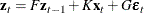and an observation equation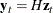where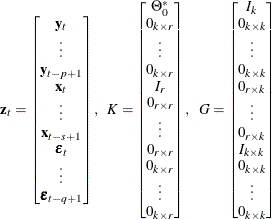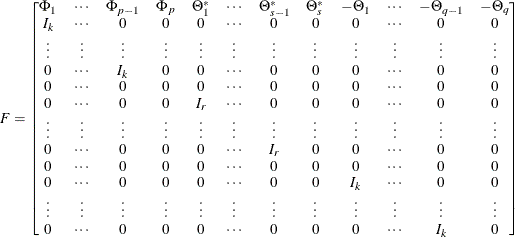and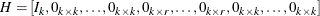On the other hand, it is assumed thatfollows a VARMA(p,q) model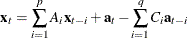The model can also be expressed as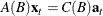whereand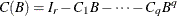are matrix polynomials in, and the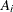and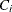arematrices. Without loss of generality, the AR and MA orders can be taken to be the same as the VARMAX(p,q,s) model, and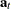andare independent white noise processes.

Under suitable conditions such as stationarity,is represented by an infinite order moving-average process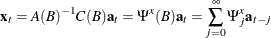where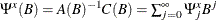.

The optimal minimum mean squared error (minimum MSE) i-step-ahead forecast of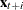is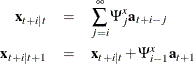For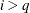,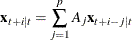The VARMAX(p,q,s) model has an absolutely convergent representation as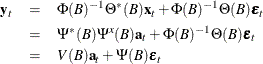or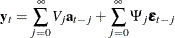where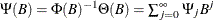,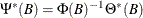, and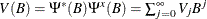.

The optimal (minimum MSE) i-step-ahead forecast of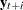is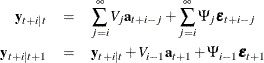for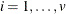with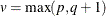. For,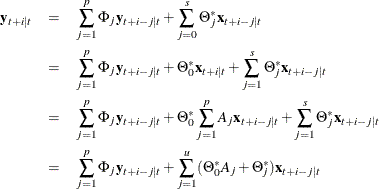where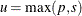.

Define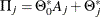. For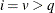with, you obtain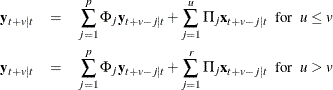From the preceding relations, a state equation is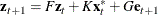and an observation equation iswhere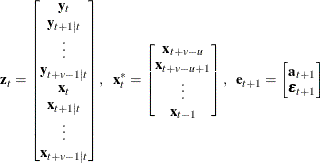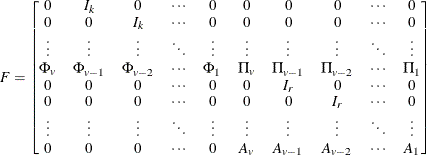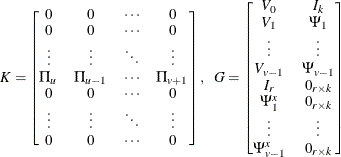and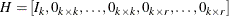Note that the matrix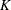and the input vector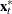are defined only when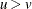.